# 27 Results

View
Selected filters:
• Trigonometry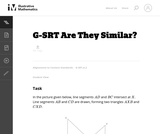Conditions of Use:
No Strings Attached
Rating

In this problem, students are given a picture of two triangles that appear to be similar, but whose similarity cannot be proven without further information. Asking students to provide a sequence of similarity transformations that maps one triangle to the other focuses them on the work of standard G-SRT.2, using the definition of similarity in terms of similarity transformations.

Subject:
Mathematics
Geometry
Trigonometry
Material Type:
Activity/Lab
Provider:
Illustrative Mathematics
Provider Set:
Illustrative Mathematics
Author:
Illustrative Mathematics
05/01/2012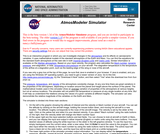Conditions of Use:
Rating

Atmosphere Applet: This program lets you study how the properties of the atmosphere change with altitude. You can study the atmosphere of either the Earth or Mars. The equations used in this program are taken from the ICAO standard day model for the Earth and from some curve fits of the Martian atmosphere gathered by the Global Surveyor spacecraft. Using the airplane graphic you can select an altitude, or you can type an altitude into the input box.

The program instantly outputs a selected property and displays the local temperature and pressure on gauges You can output the temperature, pressure, density, local speed of sound, Mach number for specified velocity, or the ratio of aircraft lift to the lift on Earth at sea level. Input and output can be given in either English or metric units.

Subject:
Engineering
Algebra
Calculus
Functions
Trigonometry
Chemistry
Geoscience
Physics
Space Science
Material Type:
Full Course
Interactive
Provider:
NASA
Provider Set:
ComPADRE: Resources for Physics and Astronomy Education
NASA Glenn Research Center
Author:
NASA Glenn Research Center
Tom Benson
08/09/2008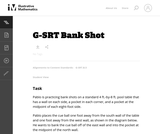Conditions of Use:
No Strings Attached
Rating

This task asks students to use similarity to solve a problem in a context that will be familiar to many, though most students are accustomed to using intuition rather than geometric reasoning to set up the shot.

Subject:
Mathematics
Geometry
Trigonometry
Material Type:
Activity/Lab
Provider:
Illustrative Mathematics
Provider Set:
Illustrative Mathematics
Author:
Illustrative Mathematics
08/21/2012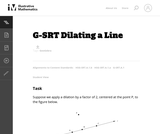Conditions of Use:
No Strings Attached
Rating

This task gives students the opportunity to verify that a dilation takes a line that does not pass through the center to a line parallel to the original line, and to verify that a dilation of a line segment (whether it passes through the center or not) is longer or shorter by the scale factor.

Subject:
Mathematics
Geometry
Trigonometry
Material Type:
Activity/Lab
Provider:
Illustrative Mathematics
Provider Set:
Illustrative Mathematics
Author:
Illustrative Mathematics
05/01/2012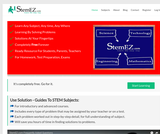Rating

Encyclopedia of STEM education is a free resource with 50,000 problems/solutions covering 13 math subjects, 13 science subjects and 13 engineering subjects for self study learning, classroom teaching, tutoring and teacher training.

Subject:
Engineering
Technology
Education
Mathematics
Algebra
Calculus
Functions
Geometry
Statistics and Probability
Trigonometry
Physical Science
Chemistry
Physics
Material Type:
Full Course
Homework/Assignment
Student Guide
Teaching/Learning Strategy
Author:
open source
12/21/2017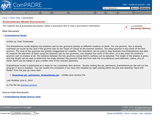Conditions of Use:
No Strings Attached
Rating

The Eratosthenes model displays the shadows cast by two gnomons (sticks) at different locations on Earth. For one gnomon, Sun is directly overhead (as would be the case if the gnomon was on the Tropic of Cancer at the summer solstice). The other gnomon is due north of the first gnomon. The sizes of the gnomons are greatly exaggerated for visibility. This simulation can be used to help illustrate how Eratosthenes was able to measure the diameter of Earth using the shadows cast by two gnomons, one situated due north of the other, on a day when the southerly gnomon cast no shadow at all. The distance between the two gnomons (along Earth's surface) can be adjusted. The length of the shadow is given, and this length can be used to determine the angle between the gnomon lines and from that the circumference (and diameter, radius, etc) of Earth. Earth can be hidden to give a better view of the relevant geometry. Eratosthenes model is distributed as a ready-to-run (compiled) Java archive. Double clicking the ejs_astronomy_Eratosthenes.jar file will run the program if Java is installed. You can modify this simulation if you have EJS installed by right-clicking within the plot and selecting "Open EJS Model" from the pop-up menu item.

Subject:
Computing and Information
Education
History, Law, Politics
Mathematics
Trigonometry
Geoscience
Physics
Space Science
Material Type:
Full Course
Interactive
Provider:
Provider Set:
ComPADRE: Resources for Physics and Astronomy Education
Author:
Todd Timberlake
01/02/2010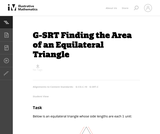Conditions of Use:
Remix and Share
Rating

This tasks examines how to calculate the area of an equilateral triangle using high school geometry.

Subject:
Mathematics
Geometry
Trigonometry
Material Type:
Activity/Lab
Provider:
Illustrative Mathematics
Provider Set:
Illustrative Mathematics
Author:
Illustrative Mathematics
01/20/2013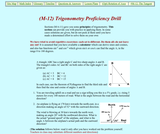Rating

This web page provides an elementary trigonometry proficiency drill. Related pages are also linked to this page. This is part of an extensive web site, "From Stargazers to Starships", that uses the topics of space exploration and space science to introduce topics in physics and astronomy.

Subject:
Education
Mathematics
Trigonometry
Physics
Material Type:
Assessment
Lecture Notes
Provider:
Provider Set:
ComPADRE: Resources for Physics and Astronomy Education
Author:
David Stern
05/14/2008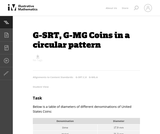Conditions of Use:
No Strings Attached
Rating

This task complements ``Seven Circles'' I, II, and III. This is a hands-on activity which students could work on at many different levels and the activity leads to many interesting questions for further investigation.

Subject:
Mathematics
Geometry
Trigonometry
Material Type:
Activity/Lab
Provider:
Illustrative Mathematics
Provider Set:
Illustrative Mathematics
Author:
Illustrative Mathematics
08/21/2012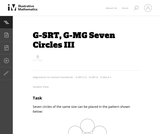Conditions of Use:
No Strings Attached
Rating

This task provides an opportunity to model a concrete situation with mathematics. Once a representative picture of the situation described in the problem is drawn (the teacher may provide guidance here as necessary), the solution of the task requires an understanding of the definition of the sine function. When the task is complete, new insight is shed on the ``Seven Circles I'' problem which initiated this investigation as is noted at the end of the solution.

Subject:
Mathematics
Geometry
Trigonometry
Material Type:
Activity/Lab
Provider:
Illustrative Mathematics
Provider Set:
Illustrative Mathematics
Author:
Illustrative Mathematics
08/21/2012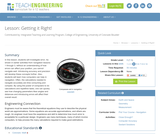Conditions of Use:
Rating

In this lesson, students will investigate error. As shown in earlier activities from navigation lessons 1 through 3, without an understanding of how errors can affect your position, you cannot navigate well. Introducing accuracy and precision will develop these concepts further. Also, students will learn how computers can help in navigation. Often, the calculations needed to navigate accurately are time consuming and complex. By using the power of computers to do calculations and repetitive tasks, one can quickly see how changing parameters likes angles and distances and introducing errors will affect their overall result.

Subject:
Engineering
Mathematics
Geometry
Trigonometry
Geoscience
Material Type:
Activity/Lab
Lesson Plan
Provider:
TeachEngineering
Provider Set:
TeachEngineering
Author:
Janet Yowell
Jeff White
Malinda Schaefer Zarske
Matt Lippis
09/18/2014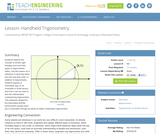Conditions of Use:
Rating

Students explore the concept of similar right triangles and how they apply to trigonometric ratios. Use this lesson as a refresher of what trig ratios are and how they work. In addition to trigonometry, students explore a clinometer app on an Android® or iOS® device and how it can be used to test the mathematics underpinning trigonometry. This prepares student for the associated activity, during which groups each put a clinometer through its paces to better understand trigonometry.

Subject:
Engineering
Mathematics
Trigonometry
Material Type:
Lesson Plan
Provider:
TeachEngineering
Provider Set:
TeachEngineering
Author:
Scott Burns
09/18/2014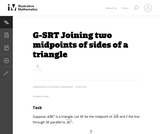Conditions of Use:
No Strings Attached
Rating

This task is closely related to very important material about similarity and ratios in geometry.

Subject:
Mathematics
Geometry
Trigonometry
Material Type:
Activity/Lab
Provider:
Illustrative Mathematics
Provider Set:
Illustrative Mathematics
Author:
Illustrative Mathematics
01/21/2013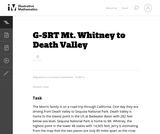Conditions of Use:
No Strings Attached
Rating

The purpose of this task is to engage students in an open-ended modeling task that uses similarity of right triangles, and also requires the use of technology.

Subject:
Mathematics
Geometry
Trigonometry
Material Type:
Activity/Lab
Provider:
Illustrative Mathematics
Provider Set:
Illustrative Mathematics
Author:
Illustrative Mathematics
02/04/2013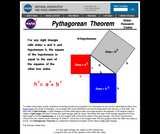Conditions of Use:
Rating

This resource features an animated Java tutorial that uses geometric overlays to show why the Pythagorean Theorem works. The resource was developed by NASA to supplement the algebraic proofs which are typically provided to students for solving problems involving right triangles. Background information on the Pythagorean Theorem and its historical use is included. This resource is part of a larger collection, developed by scientists and teacher workshop participants at NASA's Glenn Learning Research Center.

Subject:
Technology
Education
Mathematics
Trigonometry
Physics
Provider:
Provider Set:
ComPADRE: Resources for Physics and Astronomy Education
12/22/2008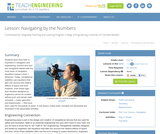Conditions of Use:
Rating

In this lesson, students will learn that math is important in navigation and engineering. Ancient land and sea navigators started with the most basic of navigation equations (Speed x Time = Distance). Today, navigational satellites use equations that take into account the relative effects of space and time. However, even these high-tech wonders cannot be built without pure and simple math concepts basic geometry and trigonometry that have been used for thousands of years. In this lesson, these basic concepts are discussed and illustrated in the associated activities.

Subject:
Engineering
Mathematics
Geometry
Trigonometry
Geoscience
Material Type:
Activity/Lab
Lesson Plan
Provider:
TeachEngineering
Provider Set:
TeachEngineering
Author:
Janet Yowell
Jeff White
Malinda Schaefer Zarske
09/18/2014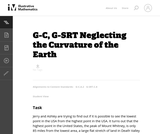Conditions of Use:
No Strings Attached
Rating

This task applies geometric concepts, namely properties of tangents to circles and of right triangles, in a modeling situation. The key geometric point in this task is to recognize that the line of sight from the mountain top towards the horizon is tangent to the earth. We can then use a right triangle where one leg is tangent to a circle and the other leg is the radius of the circle to investigate this situation.

Subject:
Mathematics
Geometry
Trigonometry
Material Type:
Activity/Lab
Provider:
Illustrative Mathematics
Provider Set:
Illustrative Mathematics
Author:
Illustrative Mathematics
03/04/2013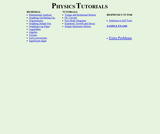Rating

A series of online tutorial units with quizzes designed for students in the introductory college or university physics course. The page includes topics from math, physics, and biophysics. Remedial material includes simple math concepts. Some of these include: dimensional analysis, trigonometry, graphing on log paper, logarithms, algebra, vectors, unit conversions and significant digits. Along with these, the tutorials introduce torque and rotational motion, DC circuits, exponent growth and decay and simple harmonic motion. The author does make note that all of these tutorials are best viewed in Internet Explorer.

Subject:
Life Science
Mathematics
Trigonometry
Physics
Material Type:
Assessment
Lecture Notes
Provider:
AMSER: Applied Math and Science Education Repository
Provider Set:
AMSER: Applied Math and Science Education Repository
ComPADRE: Resources for Physics and Astronomy Education
Author:
Bill Teesdale
Teesdale, Bill
10/10/2003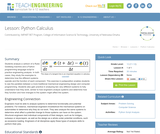Conditions of Use:
Rating

Students analyze a cartoon of a Rube Goldberg machine and a Python programming language script to practice engineering analysis. In both cases, they study the examples to determine how the different systems operate and the function of each component. This exercise in juxtaposition enables students to see the parallels between a more traditional mechanical engineering design and computer programming. Students also gain practice in analyzing two very different systems to fully understand how they work, similar to how engineers analyze systems and determine how they function and how changes to the system might affect the system.

Subject:
Computing and Information
Engineering
Education
Mathematics
Trigonometry
Material Type:
Lesson Plan
Provider:
TeachEngineering
Provider Set:
TeachEngineering
Author:
Brian Sandall
Scott Burns
09/18/2014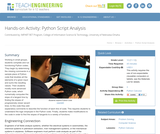Conditions of Use:
Rating

Working in small groups, students complete and run functioning Python codes. They begin by determining the missing commands in a sample piece of Python code that doubles all the elements of a given input and sums the resulting values. Then students modify more advanced Python code, which numerically computes the slope of a tangent line by finding the slopes of progressively closer secant lines; to this code they add explanatory comments to describe the function of each line of code. This requires students to understand the logic employed in the Python code. Finally, students make modifications to the code in order to find the slopes of tangents to a variety of functions.

Subject:
Computing and Information
Engineering
Education
Mathematics
Trigonometry
Material Type:
Activity/Lab
Provider:
TeachEngineering
Provider Set:
TeachEngineering
Author:
Brian Sandall
Scott Burns
09/18/2014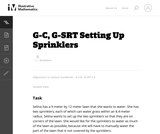Conditions of Use:
No Strings Attached
Rating

This modeling task involves several different types of geometric knowledge and problem-solving: finding areas of sectors of circles (G-C.5), using trigonometric ratios to solve right triangles (G-SRT.8), and decomposing a complicated figure involving multiple circular arcs into parts whose areas can be found (MP.7).

Subject:
Mathematics
Geometry
Trigonometry
Material Type:
Activity/Lab
Provider:
Illustrative Mathematics
Provider Set:
Illustrative Mathematics
Author:
Illustrative Mathematics
05/01/2012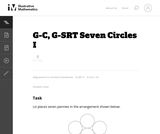Conditions of Use:
No Strings Attached
Rating

This task is intended to help model a concrete situation with geometry. Placing the seven pennies in a circular pattern is a concrete and fun experiment which leads to a genuine mathematical question: does the physical model with pennies give insight into what happens with seven circles in the plane?

Subject:
Mathematics
Geometry
Trigonometry
Material Type:
Activity/Lab
Provider:
Illustrative Mathematics
Provider Set:
Illustrative Mathematics
Author:
Illustrative Mathematics
08/20/2012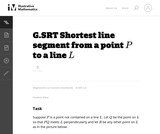Conditions of Use:
No Strings Attached
Rating

This is a foundational geometry task designed to provide a route for students to develop some fundamental geometric properties that may seem rather obvious at first glance. In this case, the fundamental property in question is that the shortest path from a point to a line meets the line at a right angle, which is crucial for many further developments in the subject.

Subject:
Mathematics
Geometry
Trigonometry
Material Type:
Activity/Lab
Provider:
Illustrative Mathematics
Provider Set:
Illustrative Mathematics
Author:
Illustrative Mathematics
12/15/2012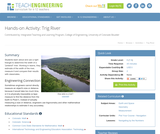Conditions of Use:
Rating

Students learn about and use a right triangle to determine the width of a "pretend" river. Working in teams, they estimate of the width of the river, measure it and compare their results with classmates.

Subject:
Engineering
Trigonometry
Material Type:
Activity/Lab
Lesson Plan
Provider:
TeachEngineering
Provider Set:
TeachEngineering
Author:
Janet Yowell
Jeff White
Malinda Schaefer Zarske
09/26/2008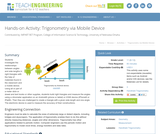Conditions of Use:
Rating

Students investigate the relationships between angles and side lengths in right triangles with the help of materials found in the classroom and a mobile device. Using all or part of a meter stick or dowel and text books or other supplies, students build right triangles and measure the angles using a clinometer application on an Android® (phone or tablet) or iOS® device (iPhone® or iPad®). Then they are challenged to create a triangle with a given side length and one angle. The electronic device is used to measure the accuracy of their constructions.

Subject:
Engineering
Mathematics
Trigonometry
Material Type:
Activity/Lab
Provider:
TeachEngineering
Provider Set:
TeachEngineering
Author:
Scott Burns
09/18/2014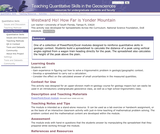Conditions of Use:
Remix and Share
Rating

PowerPoint module leading students through development of a spreadsheet to calculate the distance of a mountain peak from coplanar vertical angles shot from two points a known distance apart.

Subject:
Education
Trigonometry
Geoscience
Material Type:
Activity/Lab
Lecture Notes
Provider:
Science Education Resource Center (SERC) at Carleton College
Provider Set:
Teach the Earth
Author:
Len Vacher
08/01/2012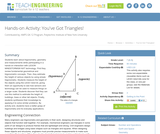Conditions of Use:
Rating

Students learn about trigonometry, geometry and measurements while participating in a hands-on interaction with LEGO® MINDSTORMS® NXT technology. First they review fundamental geometrical and trigonometric concepts. Then, they estimate the height of various objects by using simple trigonometry. Students measure the height of the objects using the LEGO robot kit, giving them an opportunity to see how sensors and technology can be used to measure things on a larger scale. Students discover that they can use this method to estimate the height of buildings, trees or other tall objects. Finally, students synthesize their knowledge by applying it to solve similar problems. By activity end, students have a better grasp of trigonometry and its everyday applications.

Subject:
Engineering
Technology
Education
Mathematics
Geometry
Trigonometry
Material Type:
Activity/Lab
Provider:
TeachEngineering
Provider Set:
TeachEngineering
Author:
Raymond Le Grand